# Nickzom Calculator Calculates Permutation and Combination With Steps – The Calculator Encyclopedia

According to Wikipedia,

In mathematics, the notion of permutation relates to the act of arranging all the members of a set into some sequence or order, or if the set is already ordered, rearranging (reordering) its elements, a process called permuting.

In mathematics, a combination is a selection of items from a collection, such that (unlike permutations) the order of selection does not matter.

Getting answers and workings (steps) for permutation and combination problems in Nickzom Calculator – The Calculator Encyclopedia is very simple and I would very much love to show you how you can do this easily.

You can access Nickzom Calculator (The Calculator Encyclopedia) via any of these channels:

First and Foremost, proceed to the Calculator Map, under the Mathematics section click on Permutation|Combination.

Let’s begin with solving a problem on Permutation. I hope by now you do know the value of n and r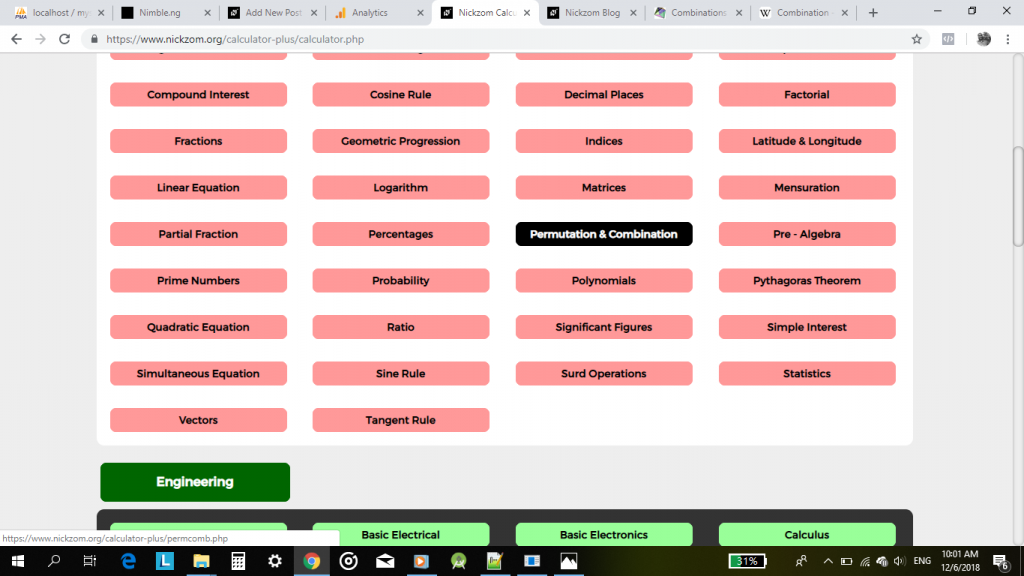Click on Permutation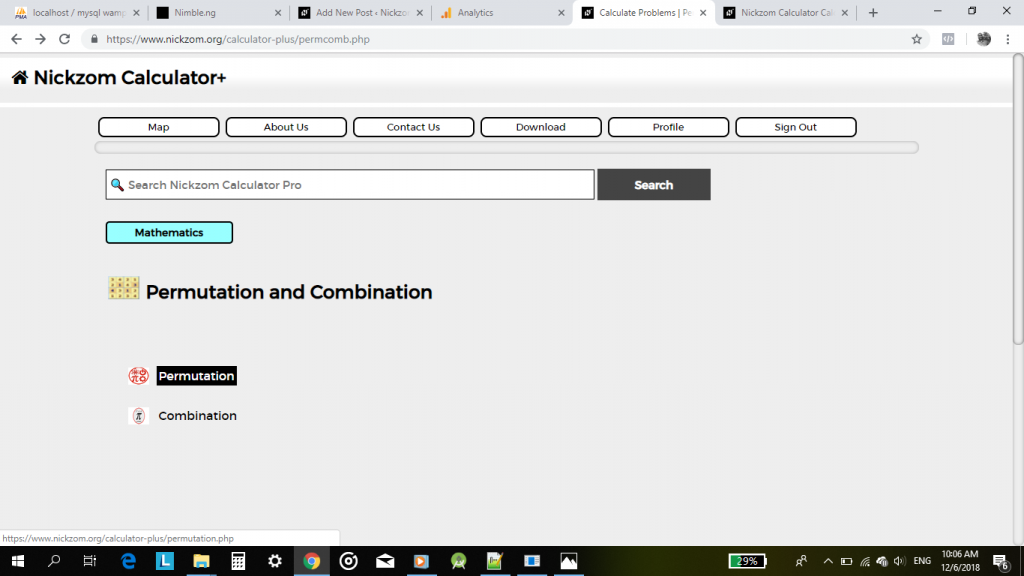An interface for you to enter your values of n and r is displayed for you.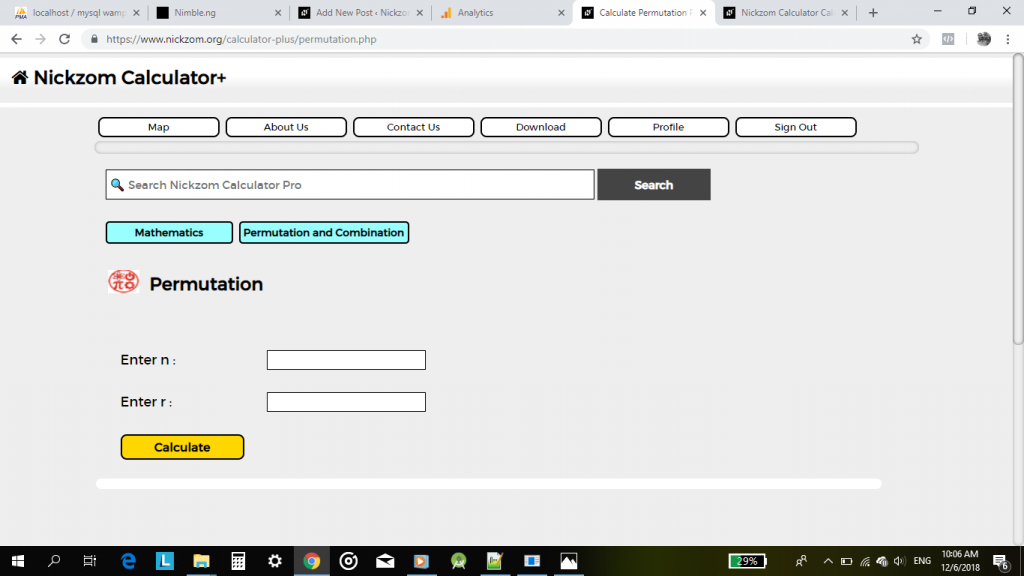Now, enter the values.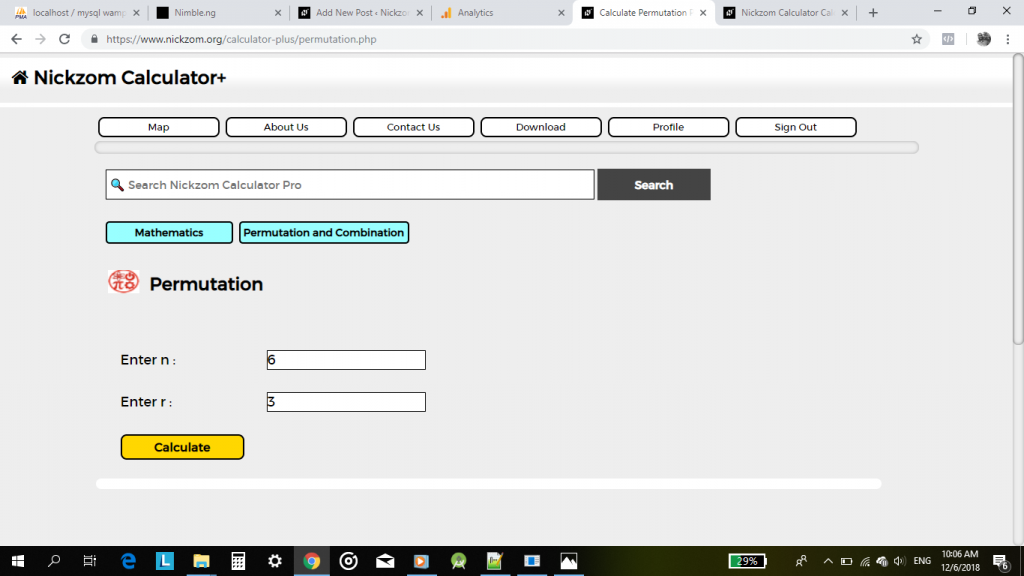Finally, click on Calculate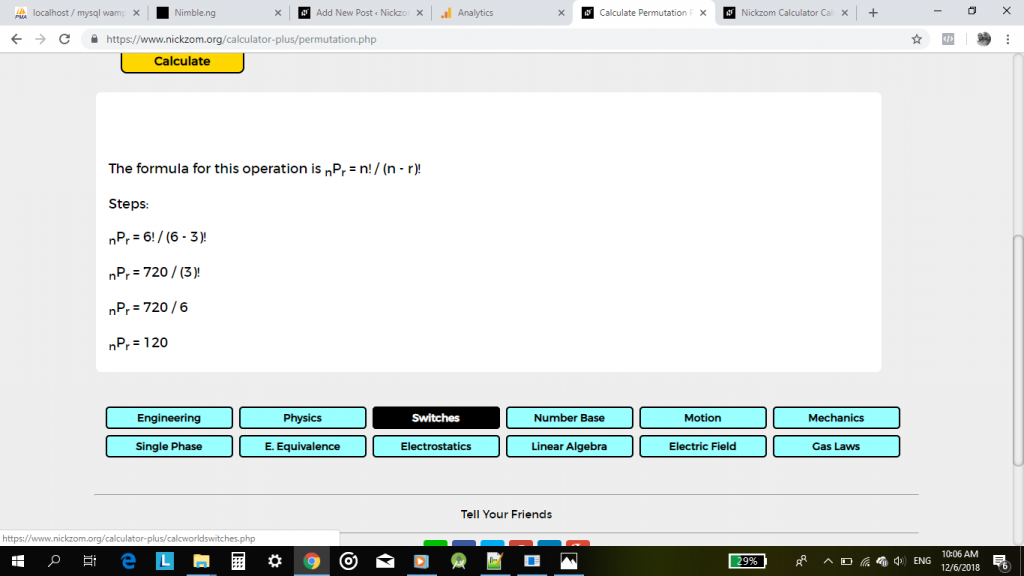As clear as crystal, the formula, workings and steps is properly displayed for you. I do hope you understand, right?

Great, moving on to Combination navigate back to the Permutation and Combination page and click on Combination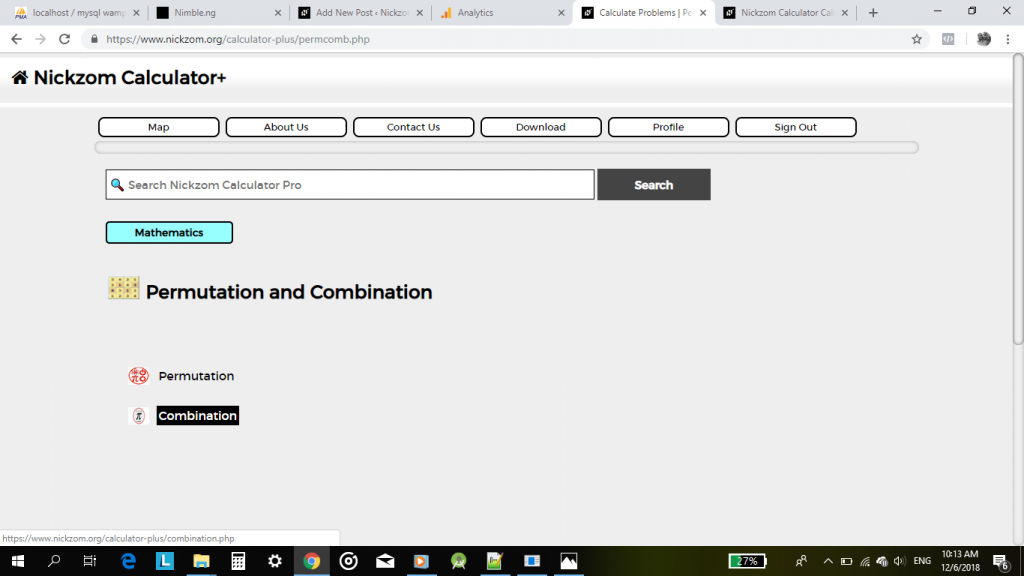The combination interface is displayed for you to enter the values.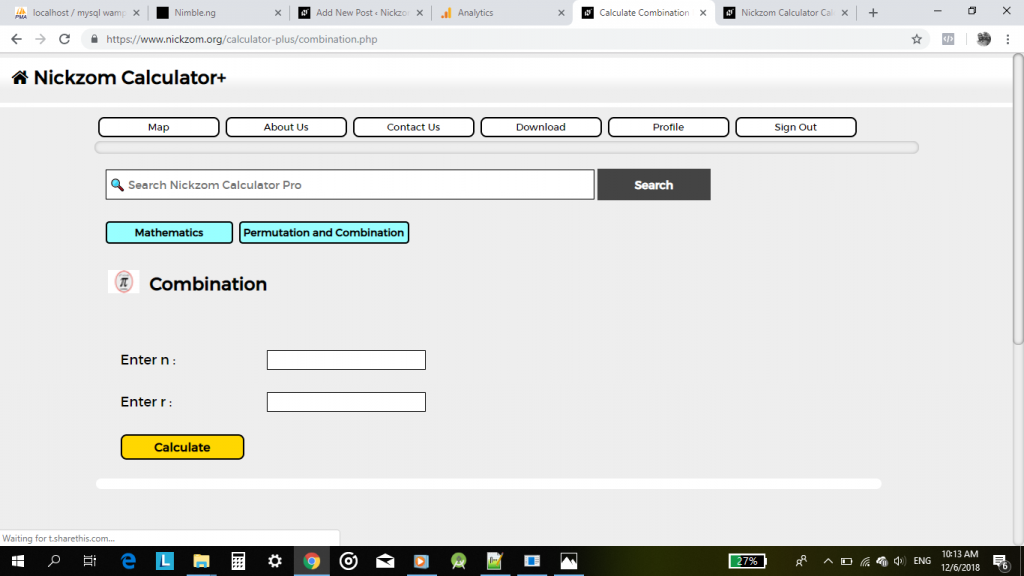Enter the accurate values according to the n and r parameters.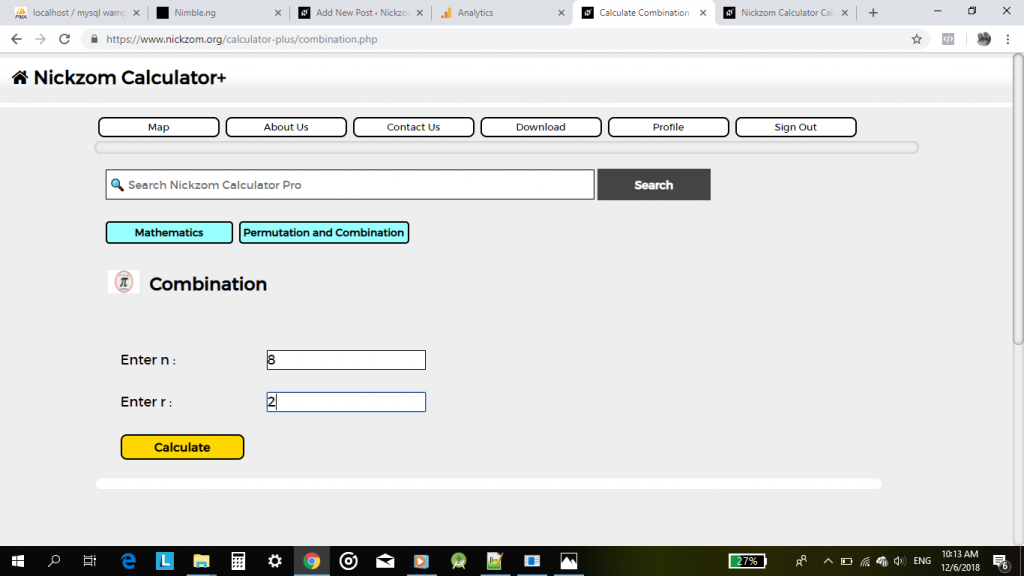Lastly, click on Calculate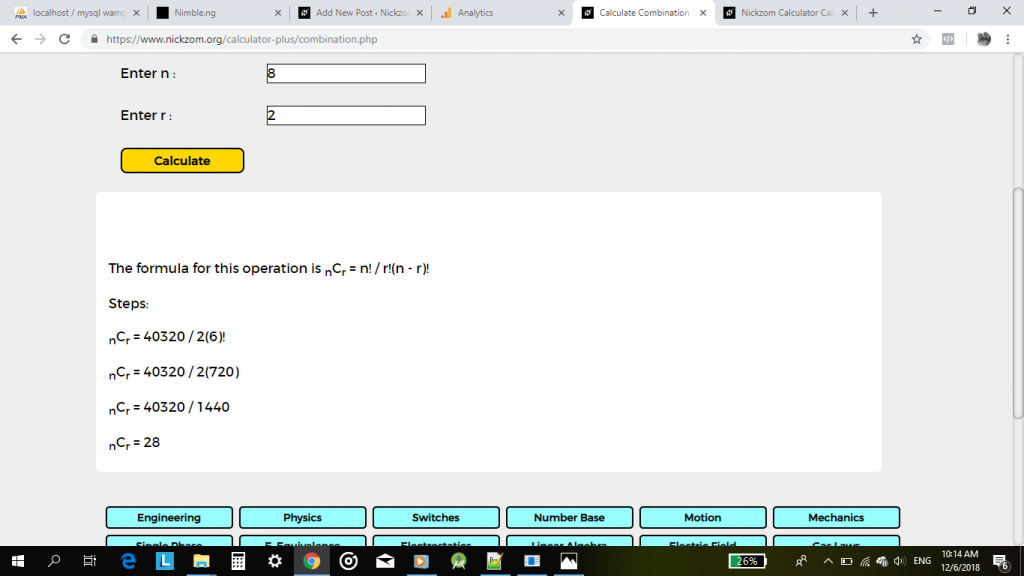Once again, a display of the result is clearly shown.

Thank you!